6.1 - 6.3 Review
starstarstarstarstarstarstarstarstarstar
by Kristen Field
| 20 Questions1
1
A
B
C
D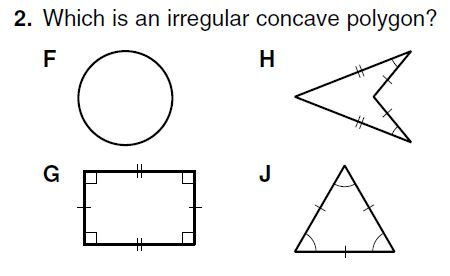2
1
F
G
H
J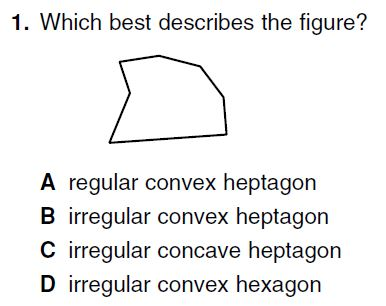3
1
A
B
C
D
4
1
Determine the measure of each interior angle of a regular dodecagon.
60
120
150
18005
1
A
B
C
D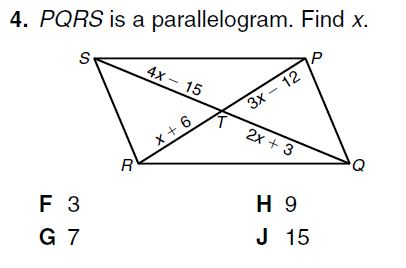6
1
F
G
H
J7
1
F
G
H
J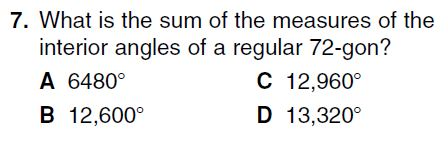8
1
A
B
C
D9
1
F
G
H
J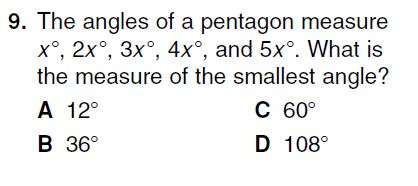10
1
A
B
C
D11
1
A
B
C
D12
1
A
B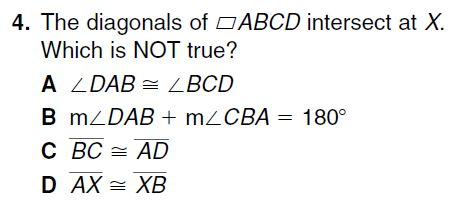13
1
A
B
C
D14
1
A
B
C
D15
1
F
G
H
J16
1
F
G
H
J17
1
A
B
C
D
18
1
On a seperate piece of paper, plot the following points: E (1, 1), F (4, 5), G (6, 6), H (3, 2). Show that the figure is a parallelogram using the definition of a parallelogram.

Check off that you completed each step.
(You have to turn this paper in before you can take your retake)
Did you plot the figure?
Did you find the slope of
Did you find the slope of GH?
Did you find the slope of FG?
Did you find the slope of EH?
Did you write your conclusion statement?
19
1
On a seperate piece of paper, plot the following points: J (-4, -1), K (-7, -4), L (2, -10), M (5, -7). Show that the figure is a parallelogram using Theorem 6-3-1, one pair of sides are parallel and congruent.

Check off that you completed each step.
(You have to turn this paper in before you can take your retake)
Did you plot the figure?
Did you find the slope of KL?
Did you find the slope of JM?
Did you use the distance formula to find the length of KL?
Did you use the distance formula to find the length of JM?
Did you write your conclusion statement?
20
1
On a seperate piece of paper, plot the following points: P (2, 2), Q (1, -3), R (-4, 2), S (-3, 7); Show that the figure is a parallelogram using Theorem 6-3-2, opposite sides are congruent.

Check off that you completed each step.
(You have to turn this paper in before you can take your retake)
Did you plot the figure?
Did you use the distance formula to find the length of PQ?
Did you use the distance formula to find the length of RS?
Did you use the distance formula to find the length of QR?
Did you use the distance formula to find the length of PS?
Did you write your conclusion statement?